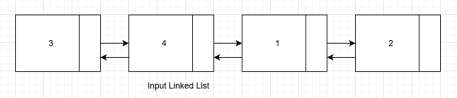# Count triplets in a sorted doubly linked list whose sum is equal to a given value x in C++

C++Server Side ProgrammingProgramming

Given a sorted doubly linked list containing integer values. The goal is to find triplets whose product is equal to the given value x. If input linked list is 3−4−1−2 and x is 6 then count will be 1 (triplet (3,1,2) )For Example

## Input

linked list: [ 3−4−13−5−10−10−0 ] x=20

## Output

Count of triplets in a sorted doubly linked list whose product is equal to a given
value x are: 2

## Explanation

Triplets will be:
( 3,4,13 ) and ( 10,10,0 )

## Input

linked list: [ 4−3−1−5−2−4−2 ] x=8

## Output

Count of triplets in a sorted doubly linked list whose product is equal to a given
value x are: 6

## Explanation

Triplets will be:
( 4,3,1), (1,5,2), (3,1,4), (1,5,2), (4,2,2) and (2,4,2),

Approach used in the below program is as follows

In this approach.

• We are taking a linked list node as struct containing int data part and self−referential next and previous pointers.

• Function Product_x(struct block* head, int x) takes the pointer to the head of doubly linked list and integer x and returns the count of triplets of nodes having product of data part as x.

• Take the initial count as 0.

• Take three pointers temp_1, temp_2 and temp_3 of type struct block.

• Starting from temp_1 pointing to the head of the linked list, temp_2 pointing to next to temp_1 and temp_3 pointing to the next to temp_3, we have three pointers pointing to the first three nodes.

• Traverse using these pointers until the last node.

• If current data parts of all above pointers have product as x. ((temp_1−>data + temp_2−>data + temp_3−>data) == x) then increment count.

• At the end we counted the number of triplets and stored in count.

• Return count as result.

## Example

Live Demo

#include <iostream>
using namespace std;
struct block{
int data;
struct block *next, *prev;
};
void insert_node(struct block** head, int data){
struct block* ptr = new block();
ptr−>data = data;
ptr−>next = NULL;
ptr−>prev = NULL;
} else {
}
}
int sum_x(struct block* head, int x){
int count = 0;
struct block *temp_1, *temp_2, *temp_3;
for (temp_1 = head; temp_1!= NULL; temp_1 = temp_1−>next){
for (temp_2 = temp_1−>next; temp_2 != NULL; temp_2 = temp_2−>next){
for (temp_3 = temp_2−>next; temp_3!= NULL; temp_3 = temp_3−>next){
if ((temp_1−>data + temp_2−>data + temp_3−>data) == x){
count++;
}
}
}
}
return count;
}
int main(){
}
Count of triplets in a sorted doubly linked list whose sum is equal to a given value x are: 1